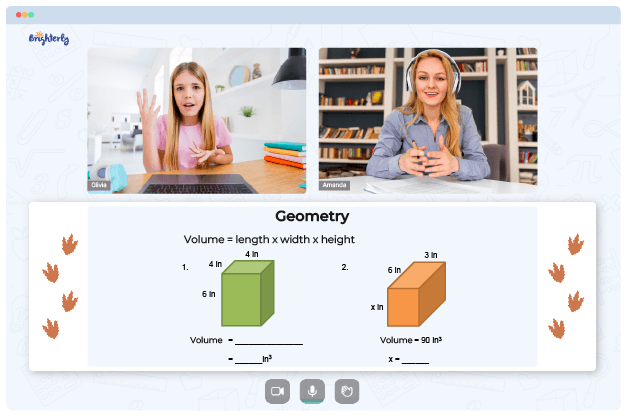# Area Model Multiplication Worksheets

Students can have trouble learning multiplication, especially if they don’t have access to the right tools and resources. The use of area model multiplication worksheets has skyrocketed in recent years. These worksheets help students improve their problem-solving abilities and comprehend the concept of multiplication by providing a visual representation of the problems.

## Worksheets on Area Model Multiplication

Multiplication area model worksheets are assets that can help students visualize the process of multiplying. It assists them in making connections between the abstract symbols and the concrete objects they represent.

Math for Kids

Is Your Child Struggling With Math?
1:1 Online Math TutoringEach factor is represented as a rectangle in the area model, breaking it into smaller parts. Next, every square shape’s length and width are compared to the elements’ digits. The region of every square shape is then determined by duplicating the length and width, and the outcomes are added together to acquire the last response.

Multiplication problems that are varying in difficulty are frequently included in area model multiplication worksheets. For example, they might begin with simple duplication issues as seen in the 2 digit by 2 digit multiplication area model worksheets, then step-by-step progress to more challenging problems, including multi-digit factors. These worksheets are intended to support learning and give students good practice opportunities.

In a classroom, multiplication using area model worksheets can be used as supporting tools during math lectures. But before introducing worksheets, teachers can use manipulatives or other visual aids to explain the idea. Students can chip away at the array area model multiplication worksheets separately or in little groups, with the teacher offering help and direction depending on the situation. At home, parents can utilize area model worksheets to enhance their youngster’s learning.

## How to Use Worksheets on Area Model Multiplication

When introducing area model multiplication, you should start with straightforward problems involving numbers with one digit. Such an approach will allow students to build confidence and better understand the concept before moving on to more challenging problems. By starting with more straightforward problems, students can master the basics of area model multiplication and develop a solid foundation for solving more complex problems in the future.

Regular practice with worksheets area model for multiplication is also essential for improving proficiency in area model multiplication. Therefore, teachers should integrate these worksheets into their regular math curriculum to help students develop their learning.

Practice builds confidence and reinforces understanding; so, the more students practice, the more proficient they become. Consistent practice also helps students retain what they have learned and apply it to more challenging problems in the future.

## The Benefits of Area Model Multiplication Worksheets

Regular practice with area model multiplication worksheets PDF can help students improve their proficiency and reinforce their learning. In addition, due to its visual nature, area model multiplication can boost students’ self-assurance when solving multiplication problems.Practicing with 3-digit by 2-digit multiplication area model worksheets requires students to think creatively about dividing a problem into smaller pieces. As a result, they can foster their powerful reasoning abilities and become better problem solvers.

Struggling with Geometry?• Is your child having difficulties with mastering geometry basics?
• An online tutor could provide the necessary support.

Does your child need additional support with understanding geometry concepts? Start studying with an online tutor.